3.NF.A.3d Compare fractions
 Name:    3.NF.A.3d Compare fractions

Multiple Choice
Identify the choice that best completes the statement or answers the question.

1.

Which symbol makes this comparison true for: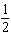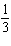a. < c. = b. >

2.

Which symbol makes this comparison true for:a. > c. = b. <

3.

Which symbol makes this comparison true for: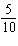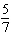a. < c. > b. =

4.

Which symbol makes this comparison true for: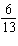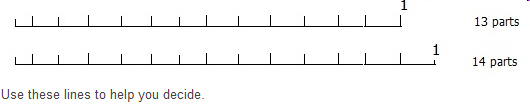a. < c. > b. =